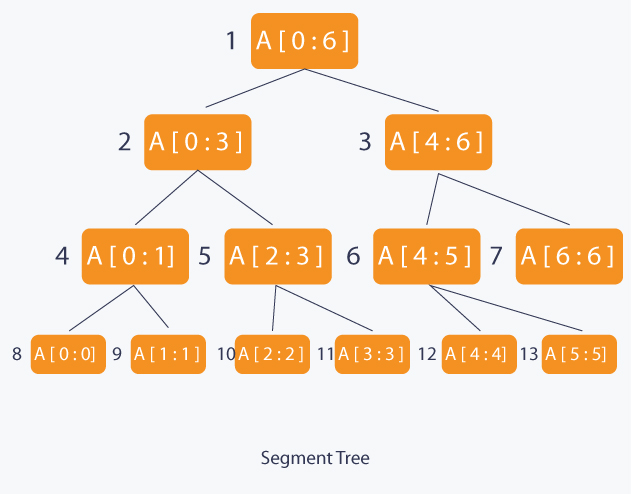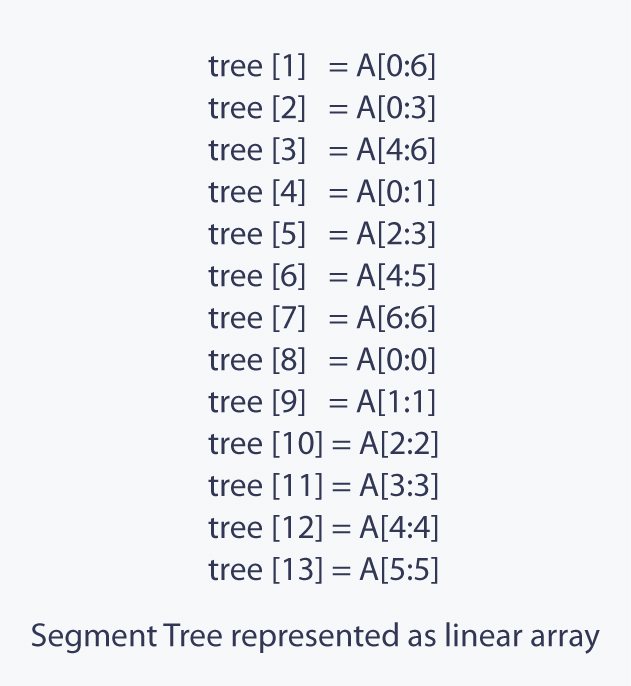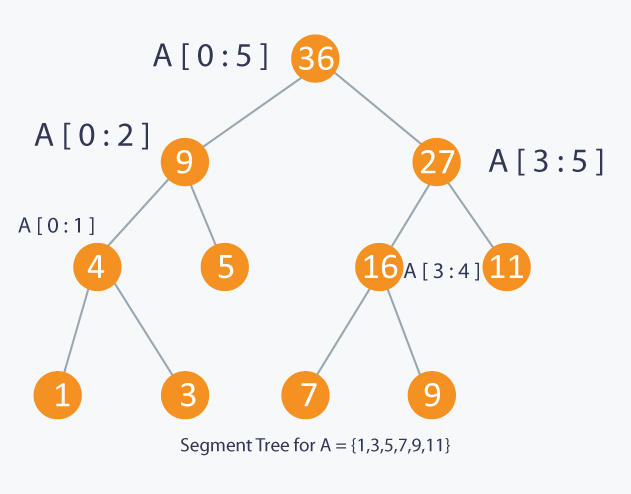349
Segment Tree and Lazy Propagation
Segment-tree
Lazy-propagation
CodeMonk
Code Monk

There are many problems in which you need to query on intervals or segments of a collection of items. For example, finding the sum of all the elements in an array from index left to right, or finding the minimum of all the elements in an array from index left to right. These problems can be easily solved with one of the most powerful data structures, Segment Tree ( in-short Segtree ).

What is Segment Tree ?
Segment tree or segtree is a basically a binary tree used for storing the intervals or segments. Each node in the segment tree represents an interval.
Consider an array A of size N and a corresponding segtree T:

1. The root of T will represent the whole array A[0:N-1].
2. Each leaf in the segtree T will represent a single element A[i] such that 0 <= i < N.
3. The internal nodes in the segtree tree T represent union of elementary intervals A[i:j] where 0 <= i < j < N.

The root of the segtree will represent the whole array A[0:N-1]. Then we will break the interval or segment into half and the two children of the root will represent the A[0:(N-1) / 2] and A[(N-1) / 2 + 1:(N-1)]. So in each step we will divide the interval into half and the two children will represent the two halves. So the height of the segment tree will be log2N. There are N leaves representing the N elements of the array. The number of internal nodes is N-1. So total number of nodes are 2*N - 1.

Once we have built a segtree we cannot change its structure i.e., its structure is static. We can update the values of nodes but we cannot change its structure. Segment tree is recursive in nature. Because of its recursive nature, Segment tree is very easy to implement. Segment tree provides two operations:

1. Update: In this operation we can update an element in the Array and reflect the corresponding change in the Segment tree.
2. Query: In this operation we can query on an interval or segment and return the answer to the problem on that particular interval.

Implementation:

Since a segtree is a binary tree, we can use a simple linear array to represent the segment tree. In almost any segtree problem we need think about what we need to store in the segment tree?.
For example, if we want to find the sum of all the elements in an array from index left to right, then at each node (except leaf nodes) we will store the sum of its children nodes. If we want to find the minimum of all the elements in an array from index left to right, then at each node (except leaf nodes) we will store the minimum of its children nodes.

Once we know what we need to store in the segment tree we can build the tree using recursion ( bottom-up approach ). We will start with the leaves and go up to the root and update the corresponding changes in the nodes that are in the path from leaves to root. Leaves represent a single element. In each step we will merge two children to form an internal node. Each internal node will represent a union of its children’s intervals. Merging may be different for different problems. So, recursion will end up at root which will represent the whole array.

For update, we simply have to search the leaf that contains the element to update. This can be done by going to either on the left child or the right child depending on the interval which contains the element. Once we found the leaf, we will update it and again use the bottom-up approach to update the corresponding change in the path from leaf to root.

To make a query on the segment tree we will be given a range from l to r. We will recurse on the tree starting from the root and check if the interval represented by the node is completely in the range from l to r. If the interval represented by a node is completely in the range from l to r, we will return that node’s value. The segtree of array A of size 7 will look like :All this we will get clear by taking an example. You can given an array A of size N and some queries. There are two types of queries:

1. Update: add a value of an element to val in the array A at a particular position idx.
2. Query: return the value of A[l] + A[l+1] + A[l+2] + ….. + A[r-1] + A[r] such that 0 <= l <= r < N

Naive Algorithm:
This is the most basic approach. For query simple run a loop from l to r and calculate the sum of all the elements. So query will take O(N). A[idx] += val will update the value of the element. Update will take O(1). This algorithm is good if the number of update operations are very large and very few query operations.

Another Naive Algorithm:
In this approach we will preprocess and store the cumulative sum of the elements of the array in an array sum. For each query simply return (sum[r] - sum[l-1]). Now query operation will take O(1). But to update, we need to run a loop and change the value of all the sum[i] such that l <= i <= r. So update operation will take O(N). This algorithm is good if the number of query operations are very large and very few update operations.

Using Segment Tree:
Let us see how to use segment tree and what we will store in the segment tree in this problem. As we know that each node of the segtree will represent an interval or segment. In this problem we need to find the sum of all the elements in the given range. So in each node we will store the sum of all the elements of the interval represented by the node. How do we do that? We will build a segment tree using recursion ( bottom-up approach ) as explained above. Each leaf will have a single element. All the internal nodes will have the sum of both of its children.

void build(int node, int start, int end)
{
if(start == end)
{
// Leaf node will have a single element
tree[node] = A[start];
}
else
{
int mid = (start + end) / 2;
// Recurse on the left child
build(2*node, start, mid);
// Recurse on the right child
build(2*node+1, mid+1, end);
// Internal node will have the sum of both of its children
tree[node] = tree[2*node] + tree[2*node+1];
}
}


In the above code we will start from the root and recurse on the left and the right child until we reach the leaves. From the leaves we will go back to the root and update all the nodes in the path. node represent the current node we are processing. Since segment tree is a binary tree. 2*node will represent the left node and 2*node + 1 represent the right node. start and end represents the interval represented by the node. Complexity of build() is O(N).To update an element we need to look at the interval in which the element is and recurse accordingly on the left or the right child.

void update(int node, int start, int end, int idx, int val)
{
if(start == end)
{
// Leaf node
A[idx] += val;
tree[node] += val;
}
else
{
int mid = (start + end) / 2;
if(start <= idx and idx <= mid)
{
// If idx is in the left child, recurse on the left child
update(2*node, start, mid, idx, val);
}
else
{
// if idx is in the right child, recurse on the right child
update(2*node+1, mid+1, end, idx, val);
}
// Internal node will have the sum of both of its children
tree[node] = tree[2*node] + tree[2*node+1];
}
}


Complexity of update will be O(logN).

To query on a given range, we need to check 3 conditions.

1. range represented by a node is completely inside the given range
2. range represented by a node is completely outside the given range
3. range represented by a node is partially inside and partially outside the given range

If the range represented by a node is completely outside the given range, we will simply return 0. If the range represented by a node is completely inside the given range, we will return the value of the node which is the sum of all the elements in the range represented by the node. And if the range represented by a node is partially inside and partially outside the given range, we will return sum of the left child and the right child. Complexity of query will be O(logN).

int query(int node, int start, int end, int l, int r)
{
if(r < start or end < l)
{
// range represented by a node is completely outside the given range
return 0;
}
if(l <= start and end <= r)
{
// range represented by a node is completely inside the given range
return tree[node];
}
// range represented by a node is partially inside and partially outside the given range
int mid = (start + end) / 2;
int p1 = query(2*node, start, mid, l, r);
int p2 = query(2*node+1, mid+1, end, l, r);
return (p1 + p2);
}


Updating an interval ( Lazy Propagation ):

Sometimes problems will ask you to update an interval from l to r, instead of a single element. One solution is to update all the elements one by one. Complexity of this approach will be O(N) per operation since where are N elements in the array and updating a single element will take O(logN) time.

To avoid multiple call to update function, we can modify the update function to work on an interval.

void updateRange(int node, int start, int end, int l, int r, int val)
{
// out of range
if (start > end or start > r or end < l)
return;

// Current node is a leaf node
if (start == end)
{
// Add the difference to current node
tree[node] += val;
return;
}

// If not a leaf node, recur for children.
int mid = (start + end) / 2;
updateRange(node*2, start, mid, l, r, val);
updateRange(node*2 + 1, mid + 1, end, l, r, val);

// Use the result of children calls to update this node
tree[node] = tree[node*2] + tree[node*2+1];
}


Let's be Lazy i.e., do work only when needed. How ? When we need to update an interval, we will update a node and mark its child that it needs to be updated and update it when needed. For this we need an array lazy[] of the same size as that of segment tree. Initially all the elements of the lazy[] array will be 0 representing that there is no pending update. If there is non-zero element lazy[k] then this element needs to update node k in the segment tree before making any query operation.

To update an interval we will keep 3 things in mind.

1. If current segment tree node has any pending update, then first add that pending update to current node.
2. If the interval represented by current node lies completely in the interval to update, then update the current node and update the lazy[] array for children nodes.
3. If the interval represented by current node overlaps with the interval to update, then update the nodes as the earlier update function

Since we have changed the update function to postpone the update operation, we will have to change the query function also. The only change we need to make is to check if there is any pending update operation on that node. If there is a pending update operation, first update the node and then work same as the earlier query function.

void updateRange(int node, int start, int end, int l, int r, int val)
{
if(lazy[node] != 0)
{
// This node needs to be updated
tree[node] += (end - start + 1) * lazy[node];    // Update it
if(start != end)
{
lazy[node*2] += lazy[node];                  // Mark child as lazy
lazy[node*2+1] += lazy[node];                // Mark child as lazy
}
lazy[node] = 0;                                  // Reset it
}
if(start > end or start > r or end < l)              // Current segment is not within range [l, r]
return;
if(start >= l and end <= r)
{
// Segment is fully within range
tree[node] += (end - start + 1) * val;
if(start != end)
{
// Not leaf node
lazy[node*2] += val;
lazy[node*2+1] += val;
}
return;
}
int mid = (start + end) / 2;
updateRange(node*2, start, mid, l, r, val);        // Updating left child
updateRange(node*2 + 1, mid + 1, end, l, r, val);   // Updating right child
tree[node] = tree[node*2] + tree[node*2+1];        // Updating root with max value
}

int queryRange(int node, int start, int end, int l, int r)
{
if(start > end or start > r or end < l)
return 0;         // Out of range
if(lazy[node] != 0)
{
// This node needs to be updated
tree[node] += (end - start + 1) * lazy[node];            // Update it
if(start != end)
{
lazy[node*2] += lazy[node];         // Mark child as lazy
lazy[node*2+1] += lazy[node];    // Mark child as lazy
}
lazy[node] = 0;                 // Reset it
}
if(start >= l and end <= r)             // Current segment is totally within range [l, r]
return tree[node];
int mid = (start + end) / 2;
int p1 = queryRange(node*2, start, mid, l, r);         // Query left child
int p2 = queryRange(node*2 + 1, mid + 1, end, l, r); // Query right child
return (p1 + p2);
}


Practice Problems: